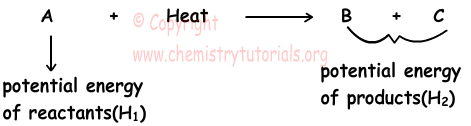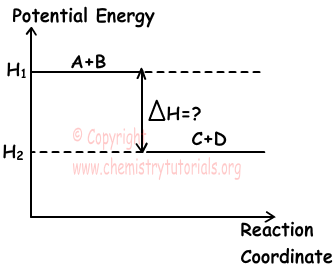## Thermochemistry

Thermochemistry

Thermochemistry deals with heat (energy) changes in chemical reactions. In chemical reactions heat is released or absorbed. If reaction absorbs heat then we call them endothermic reactions and if reaction release heat we call them exothermic reactions. Now, we examine them in detail one by one.

Endothermic Reactions:

Vaporization of water, sublimation of naphthalene, solvation of sugar in water are examples of endothermic reactions. In endothermic reactions, potential energy of reactants are lower than potential energy of products. To balance this energy difference, heat is given to reaction. Potential energy (enthalpy explained later) is shown with H.

H2O(l) + Heat → H2O(g)

Na + Heat → Na+1 + e-

2NH3 + Heat → N2 + 3H2

Look at following reaction;Graph given below shows energy changes in endothermic reactions;As you can see in the given graph, in endothermic reactions H2 is always larger than H1. Thus, ∆H=H2-H1 is always positive. In reactions, we write it like;

CaCO3(s) + Heat → CaO(s) + CO2(g) (Heat is positive)

Exothermic Reactions:

Condensation of gases, combustion reactions are examples of exothermic reactions. In these reactions, potential energies of reactants are higher than potential energies of products. Excess amount of energy is written in right side of reaction to balance energy difference.

H2O(g) → H2O(l) + Heat

O + e- → O-1 + Heat

H2(g) + 1/2O2(g) → H2O(g) + Heat

Look at following reaction;Graph given below shows energy changes in exothermic reactions;As you can see in the given graph, in exothermic reactions H1 is always larger than H2. Thus, ∆H=H2-H1 is negative. In reactions, we write it like;

N2(g) + 3H2(g) → 2NH3(g) + Heat

Example: Which ones of the following reactions are exothermic in other words ∆H is negative?

I. H2O(g) → H2O(l)               ∆H1

II. K(g) → K+(g) + e- ∆H2

III. C(s) + O2(g) → CO2(g)    ∆H3

Solution:

When matters change state from gas to liquid, they release energy. I is exothermic reaction. ∆H1 is negative.

To remove one electron from atom we should give energy, so II is endothermic reaction and  ∆H2 is positive.

In combustion reactions energy (heat) is released. III is exothermic reaction. ∆H3 is negative.

Thermochemistry Exams and  Problem Solutions

Related